×
INTELLIGENT WORK FORUMS
FOR COMPUTER PROFESSIONALS

Are you a
Computer / IT professional?
Join Tek-Tips Forums!
• Talk With Other Members
• Be Notified Of Responses
• Keyword Search
Favorite Forums
• Automated Signatures
• Best Of All, It's Free!

*Tek-Tips's functionality depends on members receiving e-mail. By joining you are opting in to receive e-mail.

#### Posting Guidelines

Promoting, selling, recruiting, coursework and thesis posting is forbidden.

# Connect two queries2

## Connect two queries

(OP)
Hi,

I have two queries:
VAC_TIME_BY_YEAR (calculates earned vacation pay)

#### CODE --> sql

SELECT dbo_TIME_T.TIME_F_EMP_NUM AS EMP_ID, dbo_TIME_T.TIME_F_DIV_NUM AS DIVISION, dbo_EE_T.EE_F_SUR AS LAST_NAME, dbo_EE_T.EE_F_FIRST AS FIRST_NAME, Sum(dbo_TIME_T.TIME_F_AMOUNT) AS EARNED, Sum([dbo_TIME_T].[TIME_F_AMOUNT])*0.04 AS VACPAY
FROM dbo_TIME_T, dbo_EE_T
WHERE (((Left([TIME_F_PAY_PERIOD],4))=[ENTER YEAR:]) AND ((dbo_TIME_T.TIME_F_DIV_NUM)=2) AND ((dbo_TIME_T.TIME_F_PAY_CODE)<499 And (dbo_TIME_T.TIME_F_PAY_CODE)<>303) AND ((dbo_TIME_T.TIME_F_EMP_NUM)=[dbo_EE_T].[EE_FP_EMP_NUM]))
GROUP BY dbo_TIME_T.TIME_F_EMP_NUM, dbo_TIME_T.TIME_F_DIV_NUM, dbo_EE_T.EE_F_SUR, dbo_EE_T.EE_F_FIRST
ORDER BY dbo_EE_T.EE_F_SUR;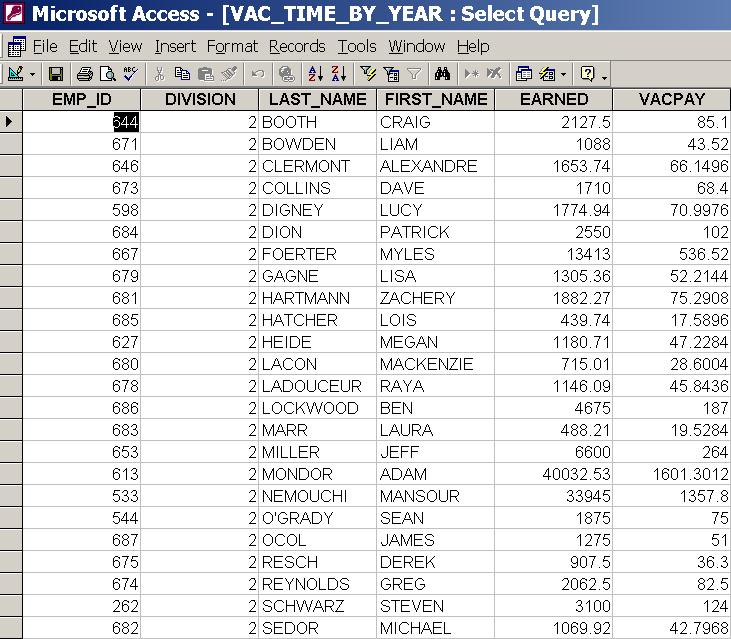and
VAC_TIME_PAID_BY_YEAR (calculates already paid vacation pay)

#### CODE --> sql

SELECT [dbo_TIME_T].[TIME_F_EMP_NUM] AS EMP_ID, [dbo_TIME_T].[TIME_F_DIV_NUM] AS DIVISION, [dbo_EE_T].[EE_F_SUR] AS LAST_NAME, [dbo_EE_T].[EE_F_FIRST] AS FIRST_NAME, Sum([dbo_TIME_T].[TIME_F_AMOUNT]) AS VAC_PAID
FROM dbo_TIME_T, dbo_EE_T
WHERE (((Left([TIME_F_PAY_PERIOD],4))=[ENTER YEAR:]) And (([dbo_TIME_T].[TIME_F_DIV_NUM])=2) And (([dbo_TIME_T].[TIME_F_PAY_CODE])=303) And (([dbo_TIME_T].[TIME_F_EMP_NUM])=[dbo_EE_T].[EE_FP_EMP_NUM]))
GROUP BY [dbo_TIME_T].[TIME_F_EMP_NUM], [dbo_TIME_T].[TIME_F_DIV_NUM], [dbo_EE_T].[EE_F_SUR], [dbo_EE_T].[EE_F_FIRST]
ORDER BY dbo_EE_T.EE_F_SUR;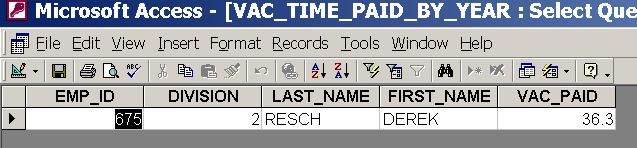I tried to combine output from both so it would show earned vacation pay, already paid vacation pay (zero if nothing paid yet), vacation pay to be paid (difference between those two), like in this Excel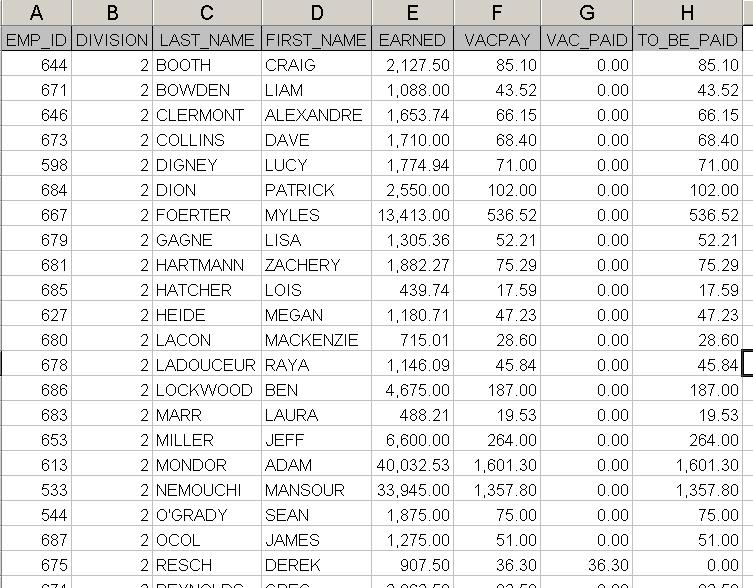All my attempts failed saying 'fields from record sources can't connect' even I created and saved relationship between those two (EMP_ID).

Is there any way to do as query (or report) instead copy and do it manually in Excel?
Thanks

### RE: Connect two queries

Normally I would do a union between the two queries. You will have to match column by column (you have an extra column in the first query that will need to be addressed). So something like this. Been a while since I have done unions in Access so, I might something amiss.

SELECT
dbo_TIME_T.TIME_F_EMP_NUM AS EMP_ID
, dbo_TIME_T.TIME_F_DIV_NUM AS DIVISION
, dbo_EE_T.EE_F_SUR AS LAST_NAME
, dbo_EE_T.EE_F_FIRST AS FIRST_NAME
, Sum(dbo_TIME_T.TIME_F_AMOUNT) AS EARNED
, Sum([dbo_TIME_T].[TIME_F_AMOUNT])*0.04 AS VACPAY
FROM dbo_TIME_T, dbo_EE_T
WHERE (((Left([TIME_F_PAY_PERIOD],4))=[ENTER YEAR:])
AND ((dbo_TIME_T.TIME_F_DIV_NUM)=2) AND ((dbo_TIME_T.TIME_F_PAY_CODE)<499 And (dbo_TIME_T.TIME_F_PAY_CODE)<>303) AND ((dbo_TIME_T.TIME_F_EMP_NUM)=[dbo_EE_T].[EE_FP_EMP_NUM]))
GROUP BY dbo_TIME_T.TIME_F_EMP_NUM, dbo_TIME_T.TIME_F_DIV_NUM, dbo_EE_T.EE_F_SUR, dbo_EE_T.EE_F_FIRST

union

SELECT [dbo_TIME_T].[TIME_F_EMP_NUM] AS EMP_ID
, [dbo_TIME_T].[TIME_F_DIV_NUM] AS DIVISION
, [dbo_EE_T].[EE_F_SUR] AS LAST_NAME
, [dbo_EE_T].[EE_F_FIRST] AS FIRST_NAME
, 0
, Sum([dbo_TIME_T].[TIME_F_AMOUNT]) AS VAC_PAID
FROM dbo_TIME_T, dbo_EE_T
WHERE (((Left([TIME_F_PAY_PERIOD],4))=[ENTER YEAR:])
And (([dbo_TIME_T].[TIME_F_DIV_NUM])=2) And (([dbo_TIME_T].[TIME_F_PAY_CODE])=303) And (([dbo_TIME_T].[TIME_F_EMP_NUM])=[dbo_EE_T].[EE_FP_EMP_NUM]))
GROUP BY [dbo_TIME_T].[TIME_F_EMP_NUM], [dbo_TIME_T].[TIME_F_DIV_NUM], [dbo_EE_T].[EE_F_SUR], [dbo_EE_T].[EE_F_FIRST]
ORDER BY dbo_EE_T.EE_F_SUR;

### RE: Connect two queries

(OP)
Hi, thanks for your input; your query runs without any error (after removing ORDER BY)

#### CODE --> sql

SELECT
dbo_TIME_T.TIME_F_EMP_NUM AS EMP_ID
, dbo_TIME_T.TIME_F_DIV_NUM AS DIVISION
, dbo_EE_T.EE_F_SUR AS LAST_NAME
, dbo_EE_T.EE_F_FIRST AS FIRST_NAME
, Sum(dbo_TIME_T.TIME_F_AMOUNT) AS EARNED
, Sum([dbo_TIME_T].[TIME_F_AMOUNT])*0.04 AS VACPAY
FROM dbo_TIME_T, dbo_EE_T
WHERE (((Left([TIME_F_PAY_PERIOD],4))=[ENTER YEAR:])
AND ((dbo_TIME_T.TIME_F_DIV_NUM)=2) AND ((dbo_TIME_T.TIME_F_PAY_CODE)<499 And (dbo_TIME_T.TIME_F_PAY_CODE)<>303) AND ((dbo_TIME_T.TIME_F_EMP_NUM)=[dbo_EE_T].[EE_FP_EMP_NUM]))
GROUP BY dbo_TIME_T.TIME_F_EMP_NUM, dbo_TIME_T.TIME_F_DIV_NUM, dbo_EE_T.EE_F_SUR, dbo_EE_T.EE_F_FIRST
union
SELECT [dbo_TIME_T].[TIME_F_EMP_NUM] AS EMP_ID
, [dbo_TIME_T].[TIME_F_DIV_NUM] AS DIVISION
, [dbo_EE_T].[EE_F_SUR] AS LAST_NAME
, [dbo_EE_T].[EE_F_FIRST] AS FIRST_NAME
, 0
, Sum([dbo_TIME_T].[TIME_F_AMOUNT]) AS VAC_PAID
FROM dbo_TIME_T, dbo_EE_T
WHERE (((Left([TIME_F_PAY_PERIOD],4))=[ENTER YEAR:])
And (([dbo_TIME_T].[TIME_F_DIV_NUM])=2) And (([dbo_TIME_T].[TIME_F_PAY_CODE])=303) And (([dbo_TIME_T].[TIME_F_EMP_NUM])=[dbo_EE_T].[EE_FP_EMP_NUM]))
GROUP BY [dbo_TIME_T].[TIME_F_EMP_NUM], [dbo_TIME_T].[TIME_F_DIV_NUM], [dbo_EE_T].[EE_F_SUR], [dbo_EE_T].[EE_F_FIRST] 

but it I am trying to add another field (column) rather than another row.
Thanks anyway.

### RE: Connect two queries

Just my \$0.02...You may consider using aliases for your tables:
FROM dbo_TIME_T T, dbo_EE_T E, it makes reading easier, IMHO

Also, since there are no spaces in your fields' names and you don't use any reserved words either (Nice!), you don't really need [] around the fields' names.

The only field that needs an indication of which table it is coming from is TIME_F_PAY_PERIOD

So your statement may just look like this:

#### CODE

SELECT
T.TIME_F_EMP_NUM AS EMP_ID
, T.TIME_F_DIV_NUM AS DIVISION
, E.EE_F_SUR       AS LAST_NAME
, E.EE_F_FIRST     AS FIRST_NAME
, Sum(T.TIME_F_AMOUNT)      AS EARNED
, Sum(T.TIME_F_AMOUNT)*0.04 AS VACPAY
FROM dbo_TIME_T T, dbo_EE_T E
WHERE (((Left(TIME_F_PAY_PERIOD,4))=[ENTER YEAR:])
AND (T.TIME_F_DIV_NUM = 2)
AND (T.TIME_F_PAY_CODE < 499 And T.TIME_F_PAY_CODE <> 303)
AND (T.TIME_F_EMP_NUM = E.EE_FP_EMP_NUM))
GROUP BY T.TIME_F_EMP_NUM, T.TIME_F_DIV_NUM, E.EE_F_SUR, E.EE_F_FIRST
union
SELECT
T.TIME_F_EMP_NUM AS EMP_ID
, T.TIME_F_DIV_NUM AS DIVISION
, E.EE_F_SUR       AS LAST_NAME
, E.EE_F_FIRST     AS FIRST_NAME
, 0
, Sum(T.TIME_F_AMOUNT) AS VAC_PAID
FROM dbo_TIME_T, dbo_EE_T
WHERE (((Left(TIME_F_PAY_PERIOD,4))=[ENTER YEAR:])
And (T.TIME_F_DIV_NUM  = 2)
And (T.TIME_F_PAY_CODE = 303)
And (T.TIME_F_EMP_NUM  = E.[EE_FP_EMP_NUM]))
GROUP BY T.TIME_F_EMP_NUM, T.TIME_F_DIV_NUM, E.EE_F_SUR, E.EE_F_FIRST 

BTW - you do know that aliases for fields matter only in first SELECT, all other fields' aliases in UNION are ignored. But it is nice to named them the same so you know which field goes where. I do the same.---- Andy

There is a great need for a sarcasm font.

### RE: Connect two queries

Good suggestions from Andrzejek but I think you're trying to get all the data into columns with joins instead of unions. If so - subqueries are your friend:

#### CODE --> sql

SELECT
dbo_EE_T.TIME_F_EMP_NUM AS EMP_ID,
dbo_EE_T.TIME_F_DIV_NUM AS DIVISION,
dbo_EE_T.EE_F_SUR AS LAST_NAME,
dbo_EE_T.EE_F_FIRST AS FIRST NAME,
n.EARNED,
n.VACPAY,
nz(m.PAID_VAC,0) as VAC_PAID
FROM
dbo_EE_T LEFT JOIN
(SELECT
dbo_TIME_T.TIME_F_EMP_NUM AS EMP_ID,
Sum(T.TIME_F_AMOUNT) AS PAID_VAC
FROM
dbo_TIME_T
WHERE
Left(TIME_F_PAY_PERIOD,4)=[ENTER YEAR:]
AND dbo_TIME_T.TIME_F_DIV_NUM = 2
AND dbo_TIME_T.TIME_F_PAY_CODE = 303
GROUP BY
dbo_TIME_T.TIME_F_EMP_NUM,
dbo_TIME_T.TIME_F_DIV_NUM) m ON dbo_EE_T.EE_F_EMP_NUM = m.TIME_F_EMP_NUM,
(SELECT
dbo_TIME_T.TIME_F_EMP_NUM AS EMP_ID,
Sum(dbo_TIME_T.TIME_F_AMOUNT) AS EARNED,
Sum(dbo_TIME_T.TIME_F_AMOUNT)*0.04 AS VACPAY,
FROM
dbo_TIME_T
WHERE
Left(TIME_F_PAY_PERIOD,4)=[ENTER YEAR:]
AND dbo_TIME_T.TIME_F_DIV_NUM = 2
AND dbo_TIME_T.TIME_F_PAY_CODE < 499
AND dbo_TIME_T.TIME_F_PAY_CODE <> 303
GROUP BY
dbo_TIME_T.TIME_F_EMP_NUM,
dbo_TIME_T.TIME_F_DIV_NUM) n
WHERE
dbo_EE_T.EE_F_EMP_NUM = n.TIME_F_EMP_NUM AND 

Note that I left joined the 'm' subquery since as you said it's possible there won't be an entry for that employee if they haven't taken any time off. This does, however, assume they've accrued some time so you might run into issues still if you run it very early in the year before any time is accrued or for new employees. I also added the Nz() around paid vacation so it will return 0 instead of null if there are no entries from the left join. I also got rid of the multiple instances of dbo_EE_T -- you don't actually need it in the subqueries, just link it in at the top level. Hope this helps.

Edit - typos in the SQL.

### RE: Connect two queries

(OP)
Thanks, zandsc1, you are right; yes, Andrzejek made valid suggestions, but I am trying to get all the data into columns with joins instead of unions.

I tried your query (with updated typo)

#### CODE --> sql

SELECT
dbo_EE_T.TIME_F_EMP_NUM AS EMP_ID,
dbo_EE_T.TIME_F_DIV_NUM AS DIVISION,
dbo_EE_T.EE_F_SUR AS LAST_NAME,
dbo_EE_T.EE_F_FIRST AS FIRST_NAME,
n.EARNED,
n.VACPAY,
nz(m.PAID_VAC,0) as VAC_PAID
FROM
dbo_EE_T LEFT JOIN
(SELECT
dbo_TIME_T.TIME_F_EMP_NUM AS EMP_ID,
Sum(T.TIME_F_AMOUNT) AS PAID_VAC
FROM
dbo_TIME_T
WHERE
Left(TIME_F_PAY_PERIOD,4)=[ENTER YEAR:]
AND dbo_TIME_T.TIME_F_DIV_NUM = 2
AND dbo_TIME_T.TIME_F_PAY_CODE = 303
GROUP BY
dbo_TIME_T.TIME_F_EMP_NUM,
dbo_TIME_T.TIME_F_DIV_NUM) m ON dbo_EE_T.EE_F_EMP_NUM = m.TIME_F_EMP_NUM,
(SELECT
dbo_TIME_T.TIME_F_EMP_NUM AS EMP_ID,
Sum(dbo_TIME_T.TIME_F_AMOUNT) AS EARNED,
Sum(dbo_TIME_T.TIME_F_AMOUNT)*0.04 AS VACPAY,
FROM
dbo_TIME_T
WHERE
Left(TIME_F_PAY_PERIOD,4)=[ENTER YEAR:]
AND dbo_TIME_T.TIME_F_DIV_NUM = 2
AND dbo_TIME_T.TIME_F_PAY_CODE < 499
AND dbo_TIME_T.TIME_F_PAY_CODE <> 303
GROUP BY
dbo_TIME_T.TIME_F_EMP_NUM,
dbo_TIME_T.TIME_F_DIV_NUM) n
WHERE
dbo_EE_T.EE_F_EMP_NUM = n.TIME_F_EMP_NUM 

but I got this error message 'Join expression not supported'

### RE: Connect two queries

Hmmm I know that Access is a bit more limited than SQL databases in terms of join capabilities but it should be able to do a simple left join like this. Your two tables have proprietary data in them so I won't ask you to post them, but can you give the SQL definitions or screen captures of them in design mode? I might be able to sleuth it out if I have those.

### RE: Connect two queries

(OP)
Yes, sure here are the screen captures; these tables are SQL Express and MS Access is used as a front end.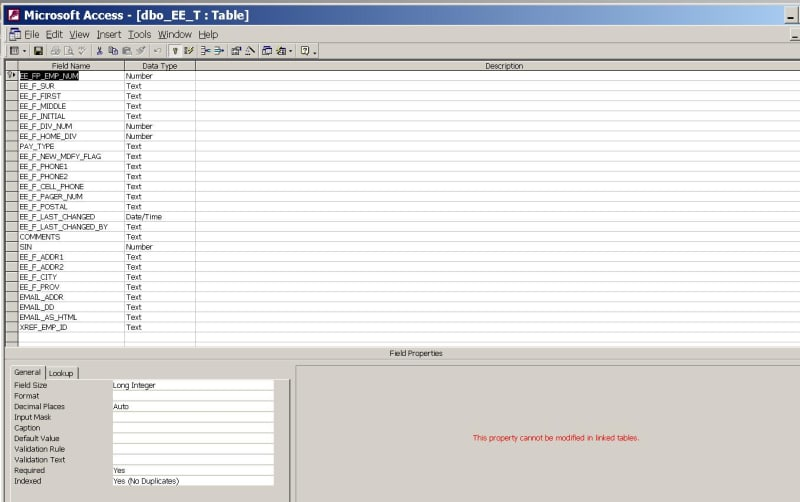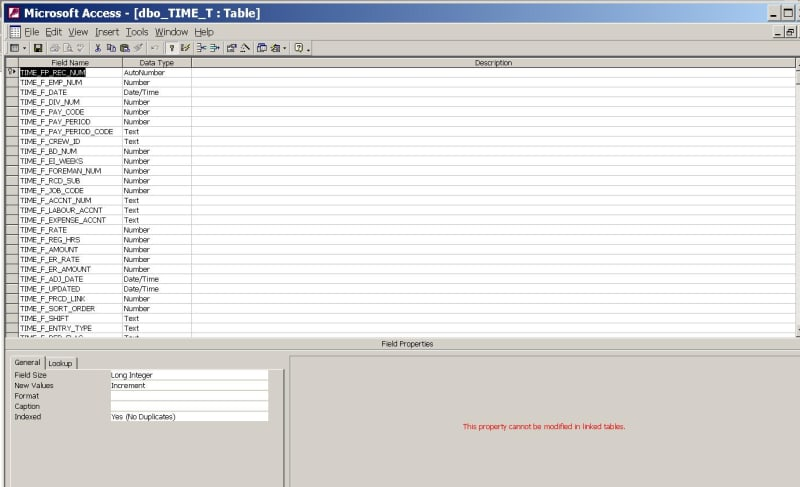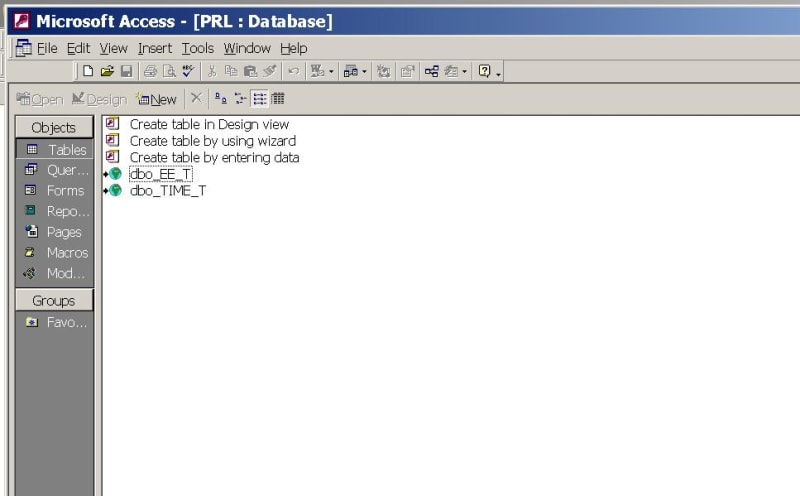Thanks

### RE: Connect two queries

Thanks. I had to nest the left join into the subquery to get it to run for some reason. This runs without error on my system. See if it gets the results you're looking for:

#### CODE --> sql

SELECT
m.EE_FP_EMP_NUM as EMP_ID,
m.EE_F_DIV_NUM as DIVISION,
dbo_EE_T.EE_F_SUR AS LAST_NAME,
dbo_EE_T.EE_F_FIRST AS FIRST_NAME,
n.EARNED,
n.VACPAY,
nz(m.PAID_VAC,0) as VAC_PAID
FROM
dbo_EE_T,
(SELECT
dbo_TIME_T.TIME_F_EMP_NUM AS EMP_ID,
dbo_TIME_T.TIME_F_DIV_NUM AS DIVISION,
Sum(dbo_TIME_T.TIME_F_AMOUNT) AS EARNED,
Sum(dbo_TIME_T.TIME_F_AMOUNT)*0.04 AS VACPAY
FROM
dbo_TIME_T
WHERE
Left(TIME_F_PAY_PERIOD,4)=[ENTER YEAR:]
AND dbo_TIME_T.TIME_F_DIV_NUM = 2
AND dbo_TIME_T.TIME_F_PAY_CODE < 499
AND dbo_TIME_T.TIME_F_PAY_CODE <> 303
GROUP BY
dbo_TIME_T.TIME_F_EMP_NUM,
dbo_TIME_T.TIME_F_DIV_NUM) n,
(SELECT
dbo_EE_T.EE_FP_EMP_NUM,
dbo_EE_T.EE_F_DIV_NUM,
sum(dbo_TIME_T.TIME_F_AMOUNT) as PAID_VAC
FROM
dbo_EE_T LEFT JOIN
dbo_TIME_T ON dbo_EE_T.EE_FP_EMP_NUM = dbo_TIME_T.TIME_F_EMP_NUM
WHERE
Left(TIME_F_PAY_PERIOD,4)=[ENTER YEAR:] AND
dbo_EE_T.EE_F_DIV_NUM=2 AND
dbo_TIME_T.TIME_F_PAY_CODE=303
GROUP BY
EE_FP_EMP_NUM,
EE_F_DIV_NUM) m
WHERE
dbo_EE_T.EE_FP_EMP_NUM = n.EMP_ID AND
n.EMP_ID = m.EE_FP_EMP_NUM 

### RE: Connect two queries

(OP)
Thank you, that's almost exactly what I try to do, except it shows only persons who already got paid right now.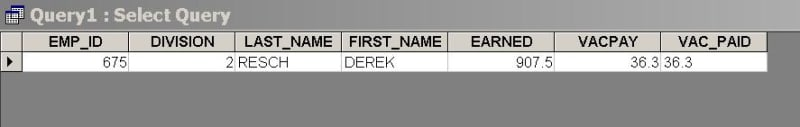### RE: Connect two queries

(OP)
It is in the format I want, but it doesn't show records if vacation not paid yet.

### RE: Connect two queries

(OP)
Well, it looks like it is working now; I did it in three queries - one for vac pay earned, one for vac pay paid and one to combine both:

Earned:

#### CODE -->

SELECT dbo_TIME_T.TIME_F_EMP_NUM AS EMP_ID, dbo_TIME_T.TIME_F_DIV_NUM AS DIVISION, dbo_EE_T.EE_F_SUR AS LAST_NAME, dbo_EE_T.EE_F_FIRST AS FIRST_NAME, Sum(dbo_TIME_T.TIME_F_AMOUNT) AS EARNED, Sum([dbo_TIME_T].[TIME_F_AMOUNT])*0.04 AS VACPAY
FROM dbo_TIME_T, dbo_EE_T
WHERE (((Left([TIME_F_PAY_PERIOD],4))=[ENTER YEAR:]) AND ((dbo_TIME_T.TIME_F_DIV_NUM)=2) AND ((dbo_TIME_T.TIME_F_PAY_CODE)<499 And (dbo_TIME_T.TIME_F_PAY_CODE)<>303) AND ((dbo_TIME_T.TIME_F_EMP_NUM)=[dbo_EE_T].[EE_FP_EMP_NUM]))
GROUP BY dbo_TIME_T.TIME_F_EMP_NUM, dbo_TIME_T.TIME_F_DIV_NUM, dbo_EE_T.EE_F_SUR, dbo_EE_T.EE_F_FIRST
ORDER BY dbo_EE_T.EE_F_SUR; 

Paid:

#### CODE -->

SELECT [dbo_TIME_T].[TIME_F_EMP_NUM] AS EMP_ID, [dbo_TIME_T].[TIME_F_DIV_NUM] AS DIVISION, [dbo_EE_T].[EE_F_SUR] AS LAST_NAME, [dbo_EE_T].[EE_F_FIRST] AS FIRST_NAME, Sum([dbo_TIME_T].[TIME_F_AMOUNT]) AS VAC_PAID
FROM dbo_TIME_T, dbo_EE_T
WHERE (((Left([TIME_F_PAY_PERIOD],4))=[ENTER YEAR:]) And (([dbo_TIME_T].[TIME_F_DIV_NUM])=2) And (([dbo_TIME_T].[TIME_F_PAY_CODE])=303) And (([dbo_TIME_T].[TIME_F_EMP_NUM])=[dbo_EE_T].[EE_FP_EMP_NUM]))
GROUP BY [dbo_TIME_T].[TIME_F_EMP_NUM], [dbo_TIME_T].[TIME_F_DIV_NUM], [dbo_EE_T].[EE_F_SUR], [dbo_EE_T].[EE_F_FIRST]
ORDER BY dbo_EE_T.EE_F_SUR; 

Show both:

#### CODE -->

SELECT [BEPAID].[EMP_ID], [BEPAID].[DIVISION], [BEPAID].[LAST_NAME], [BEPAID].[FIRST_NAME], [BEPAID].[EARNED], [BEPAID].[VACPAY], [PAID].[VAC_PAID]
FROM BEPAID LEFT JOIN PAID ON [BEPAID].[EMP_ID]=[PAID].[EMP_ID];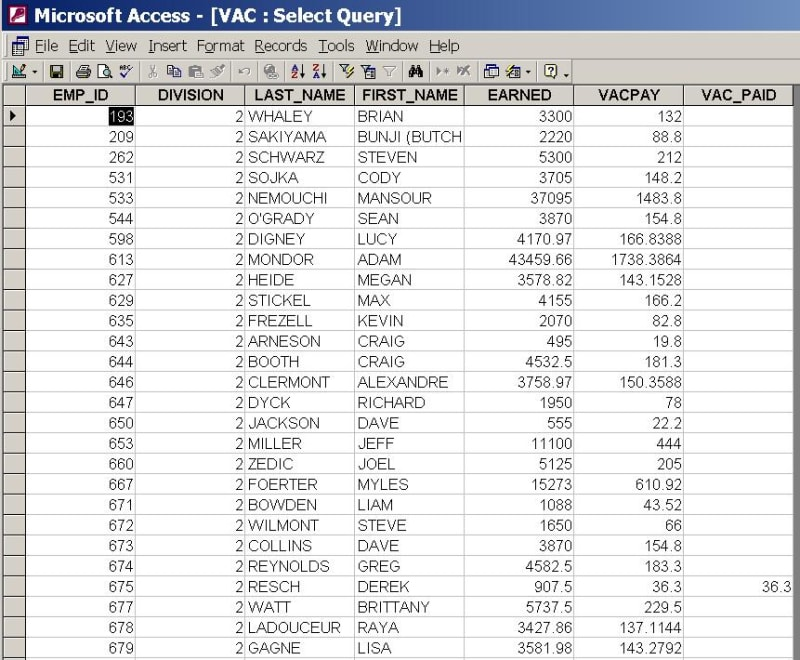It is like 90% I wanted; would be nice to show zeroes if vac pay not paid yet and add another column to the third query with 'value = earned - paid', but I will try to do it in a report.

### RE: Connect two queries

Access is doing some odd things here in terms of what it will and will not allow with left joining subqueries. I loaded up some data into tables to try and match your setup and played around with it until I got the results I wanted. This should give you everything you were asking for, including a 0 value when no vacation has been taken and a VAC_BALANCE field that is the balance between earned and taken vacation.

#### CODE --> sql

SELECT
m.EE_FP_EMP_NUM as EMP_ID,
m.EE_F_DIV_NUM as DIVISION,
dbo_EE_T.EE_F_SUR AS LAST_NAME,
dbo_EE_T.EE_F_FIRST AS FIRST_NAME,
n.EARNED,
n.VACPAY,
nz(m.PAID_VAC,0) as VAC_PAID,
n.VACPAY-nz(m.PAID_VAC,0) AS VAC_BALANCE
FROM
dbo_EE_T,
(SELECT
dbo_TIME_T.TIME_F_EMP_NUM AS EMP_ID,
dbo_TIME_T.TIME_F_DIV_NUM AS DIVISION,
Sum(dbo_TIME_T.TIME_F_AMOUNT) AS EARNED,
Sum(dbo_TIME_T.TIME_F_AMOUNT)*0.04 AS VACPAY
FROM
dbo_TIME_T
WHERE
Year(TIME_F_PAY_PERIOD)=[ENTER YEAR:]
AND dbo_TIME_T.TIME_F_DIV_NUM = 2
AND dbo_TIME_T.TIME_F_PAY_CODE < 499
AND dbo_TIME_T.TIME_F_PAY_CODE <> 303
GROUP BY
dbo_TIME_T.TIME_F_EMP_NUM,
dbo_TIME_T.TIME_F_DIV_NUM) n,
(SELECT
dbo_EE_T.EE_FP_EMP_NUM,
dbo_EE_T.EE_F_DIV_NUM,
nz(sum(dbo_TIME_T.TIME_F_AMOUNT),0) as PAID_VAC
FROM
dbo_EE_T LEFT JOIN
(SELECT * FROM dbo_TIME_T WHERE year(TIME_F_PAY_PERIOD) = [ENTER YEAR:] AND TIME_F_PAY_CODE = 303) n ON dbo_EE_T.EE_FP_EMP_NUM = n.TIME_F_EMP_NUM
WHERE
dbo_EE_T.EE_F_DIV_NUM=2
GROUP BY
EE_FP_EMP_NUM,
EE_F_DIV_NUM) m
WHERE
dbo_EE_T.EE_FP_EMP_NUM = n.EMP_ID AND
dbo_EE_T.EE_FP_EMP_NUM = m.EE_FP_EMP_NUM 

Note: I changed your LEFT(date,4) to a YEAR(date) just to be sure you would get the year value you want even if it is stored in MM/DD/YYYY instead of YYYY-MM-DD.

Note 2: the NZ(value, value if null) function is great for handling null values!

### RE: Connect two queries

(OP)
Thanks for your help; you are on the right track, it runs without error but looks like is a glitch since it returns an empty record set.

#### Red Flag This Post

Please let us know here why this post is inappropriate. Reasons such as off-topic, duplicates, flames, illegal, vulgar, or students posting their homework.

#### Red Flag Submitted

Thank you for helping keep Tek-Tips Forums free from inappropriate posts.
The Tek-Tips staff will check this out and take appropriate action.

Close Box

# Join Tek-Tips® Today!

Join your peers on the Internet's largest technical computer professional community.
It's easy to join and it's free.

Here's Why Members Love Tek-Tips Forums:

•Talk To Other Members
• Notification Of Responses To Questions
• Favorite Forums One Click Access
• Keyword Search Of All Posts, And More...

Register now while it's still free!